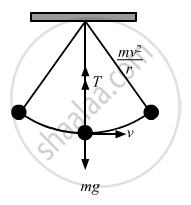Share

# The Bob of a Simple Pendulum of Length 1 M Has Mass 100 G and a Speed of 1.4 M/S at the Lowest Point in Its Path. Find the Tension in the String at this Instant. - Physics

ConceptCircular Motion

#### Question

The bob of a simple pendulum of length 1 m has mass 100 g and a speed of 1.4 m/s at the lowest point in its path. Find the tension in the string at this instant.

#### SolutionGiven:
Mass of the bob = m = 100 gm = 0.1 kg
Length of the string = r = 1 m
Speed of bob at the lowest point in its path = 1.4 m/s
Let T be the tension in the string.
From the free body diagram,

we get :

$\text{T = mg }+ \frac{\text{mv}^2}{\text{r}}$

$= \left( \frac{1}{10} \right) \times 9 . 8 + \frac{(1 . 4 )^2}{10}$

$= 0 . 98 + 0 . 196$

$= 1 . 176 \approx 1 . 2 \text{N}$

Is there an error in this question or solution?

#### APPEARS IN

Solution The Bob of a Simple Pendulum of Length 1 M Has Mass 100 G and a Speed of 1.4 M/S at the Lowest Point in Its Path. Find the Tension in the String at this Instant. Concept: Circular Motion.
S